# TIME AND WORK QUESTIONS FOR SSC EXAMS

Time and Work questions for SSC CGL, CHSL, CPO, GD, and other competitive exams. Important question from previous year exam question paper with answer and solution for upcoming examinations.

### Time and Work questions for SSC Exams

Q.1: A is thrice as efficient as B. A takes 24 days less than B to complete a work. If they work together, in how many days, will the work be completed?
(A) 15
(B) 9
(C) 8
(D) 12

Ans : (B) 9
A : B
Working capacity 3 : 1
Time 1 : 3
2 = 24
1 = 12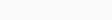$A+B=\frac{36}{4}=9$ day

Q.2: A can complete a work in 20 days, while B can complete the same work in 25 days. Both worked together for 10 days and then C alone completed the remaining work in 10 days. In how many days will A, B and C together complete the same work?
(A) 5 days
(B) 12 days
(C) 8 days
(D) 10 days

Ans : (D) 10 days

(A+B) x 10 days
9 x 10 = 90 work
left work = 10
working capacity of C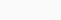$=\frac{10}{10}=1$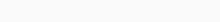$A+B+C=\frac{100}{5+4+1}=\frac{100}{10}=10$days

Q.3: A can do 60% of a work in 18 days, while B can do 40% of the same work in 10 days. Both work together for 10 days only, C completed the remaining work in 4 days. In how many days will C complete the same work?
(A) 15
(B) 25
(C) 18
(D) 12

Ans : (A) 15
A x 60% = 18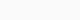$A\times\frac35=18$
A = 30 days
B x 40% = 10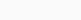$B\times\frac25=10$
B = 25 days
A = 30
B = 25
TW = 150

(A+B) x 10 = (6+5) x 10
= 110 work
left work = 150 – 110 = 40
working capacity of C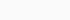$=\frac{40}{4}=10$
C alone total work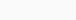$=\frac{150}{10}=15$ days

Q.4: Sachin alone can complete a piece of work for ₹8,500 in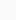$8\frac12$ days. But with the help of Vishnu, the work is completed in 6 days. The share to be paid to Vishnu is:
(A) ₹2,000
(B) ₹2,500
(C) ₹3,200
(D) ₹2,400

Ans : (B) ₹2,500
Sachin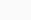$=\frac{17}{2}$ days
Sachin + Vishnu = 6 days

Share of Vishnu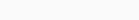$=\frac{5}{17}\times8500=2500$

Q.5: A can do a piece of work in 12 days. He worked for 6 days and left, and then B finished it in 10 days. If both work together, then in how many days will they finish the same work?
(A) 7.5
(B) 9.5
(C) 7
(D) 9

Ans : (A) 7.5
A = 12
So the work done in 6 days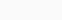$=\frac{6}{12}=\frac12$
Left work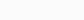$=1-\frac12=\frac12$
The left work was done by B in 10 days$B\times\frac12=10$
B = 20 day
A = 12
B = 20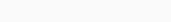$A+B=\frac{60}{5+3}=\frac{60}{8}=7.5$ day

Q.6: Suman and Lata working together can complete a task in 8 days. If Lata can complete the same task in 12 days, then how many days will Suman take to complete the same task?
(A) 10
(B) 15
(C) 18
(D) 24

Ans : (D) 24
Suman + Lata = 8
Lata = 12

Suman’ s efficiency = 3 – 2 = 1
Suman alone will complete the work$=\frac{24}{1}=24$days

Q.7: 40 persons take 6 days to complete a certain task, working 10 hours a day. How many hours a day will be sufficient for 30 persons to complete the same task in 10 days?
(A) 9
(B) 10
(C) 8
(D) 6

Ans : (C) 8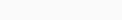$\frac{M1 T1 D1}{W1}=\frac{M2 T2 D2}{W2}$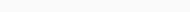$40 \times 10 \times 6 = 30 \times 10 \times X$
X = 4 x 2 = 8 day

Q.8: A can complete a task in 6 days, while B can complete the same task in 12 days. How many days will they take to complete the same task if they work together?
(A) 3
(B)$4\frac12$
(C) 5
(D) 4

Ans : (D) 4
A = 6 day
B = 12 day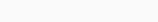$A+B =\frac{12}{2+1}=\frac{12}{3}=4$ day

Q.9: A can complete one-sixth of a work in 5 days and B can complete one-fourth of the same work in 15 days. In how many days, will both working together complete the work?
(A) 20 days
(B) 25 days
(C) 10 days
(D) 12 days

Ans : (A) 20 days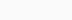$A\times\frac16=5$
A = 30 days$B\times \frac14=15$ days
B = 60 days
A = 30
B = 60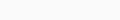$A+B =\frac{60}{3}=20$ days

Q.10: 10 men or 15 women can complete a work in 30 days. In how many days can 15 men and 27 women complete the work?
(A)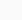$9\frac{1}{11}$
(B)$5\frac{1}{11}$
(C)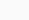$11\frac{1}{11}$
(D)$6\frac{1}{11}$

Ans : (A)$9\frac{1}{11}$
10 M = 15 M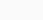$\frac{M}{F} =\frac32$
Men = M
Women = F
10 M x 30 =(15M+27F) x X
10 x 3 x 30 =(15 x 3 + 27 x 2) x X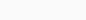$X =\frac{10\times3\times30}{99}$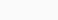$X=9\frac{1}{11}$ day

Time and Work questions for SSC Exams : Practice Set

Q.11: 15 persons working 6 hours per day earn ₹900. How much money will 20 persons earn, working 10 hours per day?
(A) ₹1,800
(B) ₹2,000
(C) ₹1,500
(D) ₹2,100

Ans : (B) ₹2,000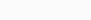$\frac{M1 T1}{R1}=\frac{M2 T2}{R2}$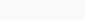$\frac{15\times6}{900}=\frac{20\times10}{x}$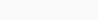$X =\frac{900\times20\times10}{15\times6}$
X = 2000₹

Q.12: Working together A, B and C can complete a piece of work in 3 days. A complete the same work in 24 days and B completes it in 6 days. How many days will C alone take to complete the same work?
(A) 12
(B) 18
(C) 8
(D) 6

Ans : (C) 8
A+B+C = 3
A = 24
B = 6

C’s efficiency = 8 -(1+4)
= 8 – 5 = 3
C alone will work$=\frac{24}{3}=8$Days

Q.13: X can complete half of the work in 20 days and Y can do one-fifth of the same work in 10 days. X started the work and left after 8 days. Then Y took over to complete the remaining work. The total number of days taken by them to complete the work is:
(A) 50 days
(B) 60 days
(C) 40 days
(D) 48 days

Ans : (D) 48 days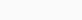$X\times\frac12=20$
X = 40 days$Y\times\frac15=10$
Y = 50 days

X worked for 8 days
5 x 8 = 40 work
Left work = 200 – 40 = 160
Remaining work by Y$=\frac{160}{4}=40$days
Total work = 40 days + 8 days = 48 days

Q.14: A completes 20% of a work in 5 days and B completes one-third of the work in 5 days. They began the work together but B leaves after 3 days. The total number of days needed for completion of the work is:
(A) 30
(B) 18
(C) 20
(D) 24

Ans : (C) 20$A\times\frac15=5$
A = 25 days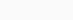$B\times\frac13=5$
B = 15 days

(A+B) Work for 3 days (A+B) x 3
16 x 3 = 48
Left work = 150 – 48 = 102
A will do the left work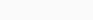$A=\frac{102}{6}=17$ days
Total work = 17+3=20 days

Q.15: Varun and Gaurav can dig a pond in 10 days and 18 days, respectively. After finishing that work together, they receive an amount of ₹7,280. What is the share of Gaurav in that amount?
(A) ₹2,600
(B) ₹2,800
(C) ₹2,700
(D) ₹2,500

Ans : (A) ₹2,600
V = 10 days
G = 18 days

Share of Gourav$=\frac{5}{14}\times7280$
= 5 x 520 = 2600 ₹

Q.16: A and B can complete a piece of work in 27 days, respectively. They completed the work in 9 days with the help of C. How many days will C take to complete eight-ninths of the work alone?
(A) 24
(B) 45
(C) 16
(D) 30

Ans : (C) 16
A = 27 days
B = 54 days
A+B+C = 9 days

C’s efficiency = 6 – (1+2)
= 6 – 3 = 3
C’s time$=\frac{54\times8}{9\times3}=16$ days

Q.17: Anu can complete a piece of work in 22 days. shama is 60% more efficient than Anu. How many days does Shama alone take to complete the same piece of work?
(A)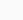$13\frac34$
(B)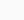$35\frac13$
(C)$36\frac23$
(D)$13\frac15$

Ans : (A)$13\frac34$
Anu = 22 days
A : S
Efficiency 100 : 160
5 : 8
Total work = 22 x 5 = 110
Shama will work alone$=\frac{110}{8}=13\frac34$ days

Thanks for attempt Time and Work questions for SSC CGL, CHSL, CPO, GD, and other competitive exams.

Time and Work questions for SSC Exams in Hindi : समय और काम प्रतियोगी परीक्षा प्रश्न :

## Maths Practice Set for Competitive Exams

Simple and Compound Interest Questions with Answers

Time and Work Questions with Answers